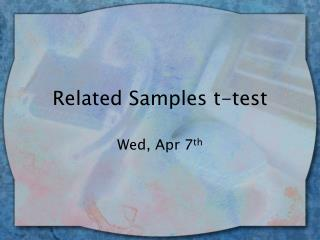DownloadDownload PresentationRelated Samples t-test

# Related Samples t-test

Télécharger la présentation## Related Samples t-test

- - - - - - - - - - - - - - - - - - - - - - - - - - - E N D - - - - - - - - - - - - - - - - - - - - - - - - - - -
##### Presentation Transcript

1. Related Samples t-test Wed, Apr 7th

2. Related Samples t • Use when: • 1 group of subjects is tested more than once (e.g., pre-test / post-test)…or • 2 groups of related (or matched) subjects are measured once (e.g., twins) • Uses same basic t formula, but focus now on ‘difference’ scores • Differences in pre- and post-test or difference in each partner in a pair

3. Hypotheses • Null hyp (Ho) indicates no difference (or no effect), so we’ll hypothesize the mean difference in the pop (D) = 0 • Ha indicates there will be a difference (2-tailed, D not = 0) or there will be a difference in a specific direction (1-tailed, D > 0 or D < 0). • We’ll reject Ho if | t obs | > | t critical| (t observed is in critical region)

4. Ex: What is the effectiveness of a new program to reduce litter? Measured aver litter in 4 cities in ’01 and ‘02

5. Find D (difference score) for each city, and then the average D (D bar) D bar = 20/4 = 5

6. Next, sum up the deviation scores (D) • Then find squared deviation scores for each city (D2) – add a new column • Then sum up the squared deviation scores, (D2) Here, D = 20, D2 = 150 • Use the T observed formula:

7. T formula for Related Samples Note: D always = 0 • T observed = (Dbar - D) SDbar Where SDbar (std error) = sqrt (SD2/ N) and SD2 = [D2 – (D)2] / N-1 = 150 – (20)2 / 3 = -83.33 SDbar = sqrt (-83.33 / 4) = -4.56 • T obs = (5-0) / -4.56 = -1.09

8. Finding T critical • Use the t table as before, • Need alpha level & 1 or 2-tailed test? • Need Df = N-1 (here N=pairs of scores or total # participants if repeated measures) • Ex) use  = .01, 1-tailed test (expected litter to decrease), Df= 3 T critical = -4.541 T observed = -1.09 So fail to reject Ho (|t obs| < |t critical|) There is no difference in litter before & after new system  not effective

9. SPSS • GSS98 dataset example… • Analyze  Compare Means  Paired Samples t-test • Pop-up window, select ‘wife…’ for var1, ‘children…’ for var2, then click arrow to put them in “Paired vars” box  OK

10. (cont.) • In output, 1st section is “Paired Samples Stats”, look for means for ‘wife…” and ‘children’ – this is what we’re comparing • In 3rd section, “Paired Samples Test”, note mean difference score, t observed, df, and ‘sig (2-tail)’. • Mean difference score is compared to 0 • Sig (2 tail) should be compared to alpha level (e.g., .05). If ‘sig’ value < alpha  reject Null • Draw a conclusion about the pre/post test scores.